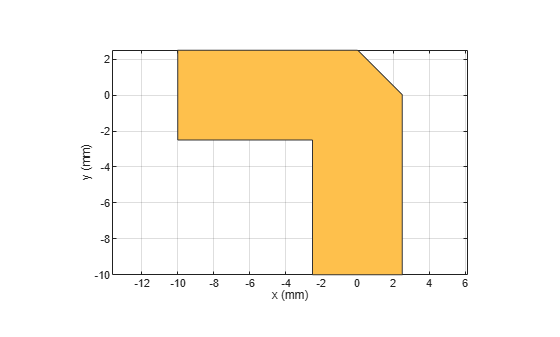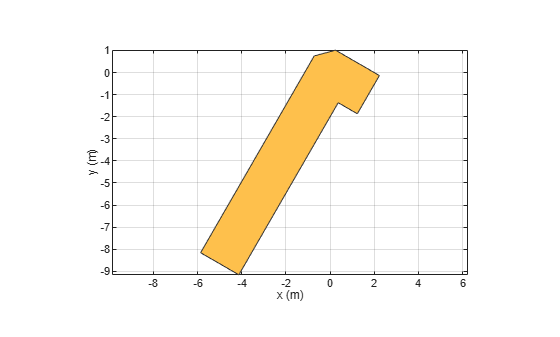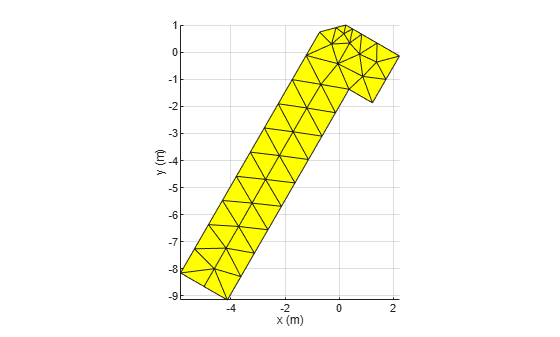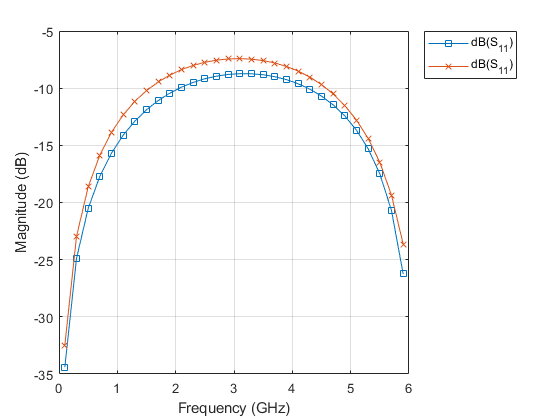# bendMitered

Create mitered bend shape on X-Y plane

Since R2021b

## Description

Use the `bendMitered` object to create a mitered bend shape on the X-Y plane.

Note

This shape object supports behavioral modeling. For more information, see Behavioral Models.

## Creation

### Syntax

``bend = bendMitered``
``bend = bendMitered(Name=Value)``

### Description

example

````bend = bendMitered` creates a mitered bend shape on the X-Y plane.```

example

````bend = bendMitered(Name=Value)` sets Properties using one or more name-value arguments. For example, `bendMitered(ReferencePoint=[1 1])` creates a mitered bend shape with the reference point at `[1 1]`. Properties not specified retain their default values.```

## Properties

expand all

Name of the mitered bend shape, specified as a character vector or a string scalar.

Example: `bend = bendMitered(Name="bendmitered1")`

Data Types: `char`

Reference point for the mitered bend shape in Cartesian coordinates, specified as a two-element vector.

Example: `bend = bendMitered(ReferencePoint=[1 2])`

Data Types: `double`

Length of the mitered bend shape in meters, specified as a two-element vector.

Example: `bend = bendMitered(Length=[0.005 0.005])`

Data Types: `double`

Width of the mitered bend shape in meters, specified as a two-element vector.

Example: `bend = bendMitered(Width=[1 1])`

Data Types: `double`

Length of the miter diagonal in meters, specified as a positive scalar.

Example: `bend = bendMitered(MiterDiagonal=2)`

Data Types: `double`

## Object Functions

 `add` Boolean unite operation on two RF PCB shapes `and` Shape1 & Shape2 for RF PCB shapes `area` Calculate area of RF PCB shape in square meters `intersect` Boolean intersection operation on two RF PCB shapes `mesh` Change and view mesh properties of metal or dielectric in PCB component `minus` Shape1 - Shape2 for RF PCB shapes `plus` Shape1 + Shape2 for RF PCB shapes `rotate` Rotate RF PCB shape about defined axis `rotateX` Rotate RF PCB shape about x-axis `rotateY` Rotate RF PCB shape about y-axis and angle `rotateZ` Rotate RF PCB shape about z-axis `subtract` Boolean subtraction operation on two RF PCB shapes `scale` Change size of RF PCB shape by fixed amount `show` Display PCB component structure or PCB shape `translate` Move RF PCB shape to new location

## Examples

collapse all

Create a mitered bend with default properties.

`bend = bendMitered`
```bend = bendMitered with properties: Name: 'myMiteredbend' ReferencePoint: [0 0] Length: [0.0100 0.0100] Width: [0.0050 0.0050] MiterDiagonal: 0.0035 ```

View the shape.

`show(bend)`Create a mitered bend shape of lengths of 10 m and 2 m, width of 2 m, and rotate it about the Z-axis by 60 degrees.

```bend = bendMitered(Length=[10 2],Width=[2 2],MiterDiagonal=1); bend = rotateZ(bend,60)```
```bend = bendMitered with properties: Name: 'myMiteredbend' ReferencePoint: [0 0] Length: [10 2] Width: [2 2] MiterDiagonal: 1 ```
`show(bend)`Mesh the mitered bend shape at a maximum edge length of 1 m.

`mesh(bend,MaxEdgeLength=1)`Create mitered bend microstrip.

```m = design(microstripLine,6e9,"Z0",75); layer2d = bendMitered('Length',[m.Length/2 m.Length/2],... "Width",[m.Width m.Width],'MiterDiagonal',sqrt(2)*m.Width); robj = pcbComponent(layer2d); robj.BoardThickness = m.Substrate.Thickness; robj.Layers{2} = m.Substrate; show(robj)```Compute and plot s-parameters.

```freq = (1:2:60)*100e6; Sckt = sparameters(robj,freq,'Behavioral',true); Sem = sparameters(robj,freq); rfplot(Sckt,1,1,'db','-s') hold on rfplot(Sem,1,1,'db','-x')```Reference:

M. Kirschning, R. H. Jansen and N. H. L. Koster, "Measurement and Computer-Aided Modeling of Microstrip Discontinuities by an Improved Resonator Method," 1983 IEEE MTT-S International Microwave Symposium Digest, Boston, MA, USA, 1983, pp. 495-497, doi: 10.1109/MWSYM.1983.1130959.

## Version History

Introduced in R2021b